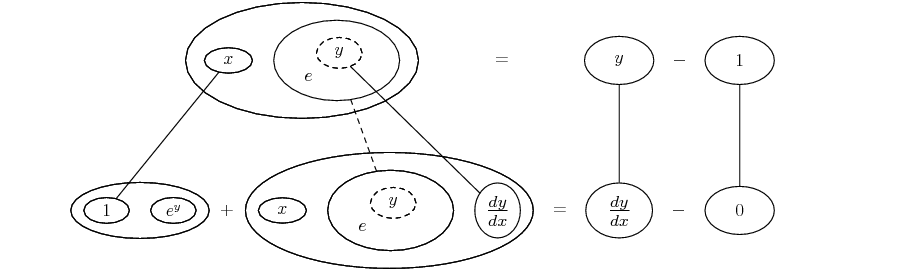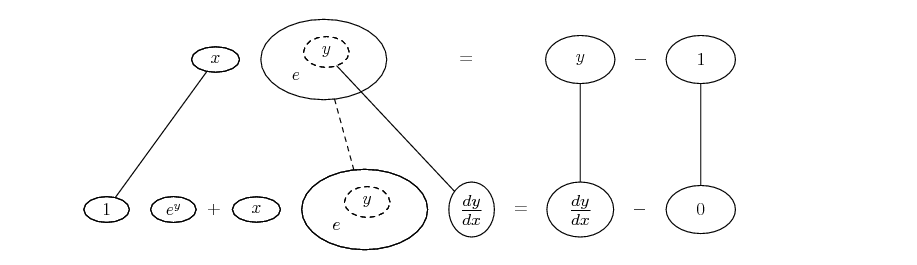# Thread: implicit differentiation to tangent line

1. ## implicit differentiation to tangent line

use implicit differentiation to find the equation of the tangent line to the curve given by equation xe^y = y-1 at the point where y=1

i know i'm supposed to implicit the equation and what i get is:

xe^y*y' = 1+y'

this is where i'm stuck, and not sure how to proceed with what i have

2. The next step would be to solve the equation for y' for finding the slope of the tangent - but you need to look again at your differentiating. You've got the implict bit, using the chain rule, but you need the product rule outside of that...

3. i'm a little confused now. do i apply the product rule before or after chain? and if it comes after, do i product rule the entire expression of e^y*y' and x or do i leave the y' seperate?

4. Picture on its way, but basically x and e^y are the two parts of the product which need the product rule. So you get x' times e^y plus x times (e^y)'

(e^y)' is the bit you've already applied the chain rule to (correctly) but then just multiplied by x (instead of applying the needed product rule)

5. ah i understand. it should be

xe^y*y' + e^y = 1+y' correct?

after applying the product rule and the chain rule that's what i got

and if i proceed to simplify it for y', i'm getting

y' = 1/(xe^y+e^y)

is that right so far?

6. Good on the left, check the right

Edit:

The pic, just in case it helps...... or...... according to taste, where... is the chain rule, wrapped here inside the legs-uncrossed version of...... the product rule. Straight continuous lines differentiate downwards (integrate up) with respect to x, and the straight dashed line similarly but with respect to the dashed balloon expression (the inner function of the composite which is subject to the chain rule).

__________________________________________

Don't integrate - balloontegrate!

http://www.ballooncalculus.org/examples/gallery.html

http://www.ballooncalculus.org/asy/doc.html

7. ah, it's supposed to be simply y' only?

seeing as how derivative of y-1 -> 1*dy/dx = y'

if that's the case, it turns out to be
y' = xe^y+e^y
y' = xe^2y

am i doing this right?

8. Nearly, but along the way you've dropped the

*y'

which you had got (correctly) from applying the chain rule

9. in getting y' = xe^2y

i subtracted the y' from the right to get xe^y*y' + e^y - y' = 0
factored out the y' to get y' = xe^y+e^y and simplified for y' = xe^2y

or is it supposed to be e^yy’? if that's the case, the problem would change considerably

10.Originally Posted by deltemisin getting y' = xe^2y

i subtracted the y' from the right to get xe^y*y' + e^y - y' = 0 Fine, but then subtract also e^y from both sides before factorising the left

factored out the y' to get y' = xe^y+e^y and simplified for y' = xe^2y

or is it supposed to be e^yy? if that's the case, the problem would change considerably
............................

11. yeah ignore that last line, not too fond of this implicit problem

i'm getting

y' = -e^y/xe^y
y' = -y ln e - xy ln e
y' = -y - xy

what am i doing wrong here?

12. Present your algebra line by line from

xe^y*y' + e^y - y' = 0

(as opposed to jumping straight to your alleged y' = -e^y/xe^y) ... and you'll probably see your error

13. xe^y*y' + e^y - y' = 0

subtract e^y from both sides to get

xe^y*y' - y' = -e^y

this is where i made my mistake, can't factor y' out like i did before

and honestly from there, i'm lost as to where to go next

14. next step is

y'(xe^y - 1) = -e^y

15. ooh, i forgot the -1 when you factor out

y'(xe^y - 1) = -e^y

divide (xe^y - 1):

y' = -e^y/(xe^y - 1)

y = 1 if and only if x = 0 so

y' = e

y = mx + b

m = e
b = 1 on account of y = 1

so tangent line equation is

y=ex+1

did i do that right?

#### Search Tags

differentiation, implicit, line, tangent